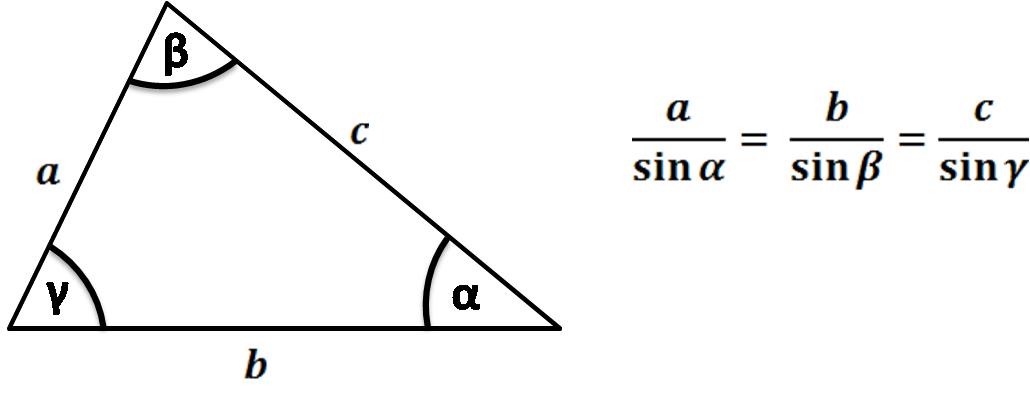# Law of Sines Calculator

Created by Bogna Szyk and Hanna Pamuła, PhD candidate
Reviewed by Steven Wooding
Last updated: Jun 21, 2022

This law of sines calculator is a handy tool for solving problems that include lengths of sides or angles of a triangle. We will explain the law of sines formula and give you a list of cases in which this rule can be deemed useful. Thanks to this triangle calculator, you will now be able to solve some trigonometry problems (more elaborate than using the Pythagorean theorem).

However, if you don't know what the sine is, first check out our sine calculator.

Prefer watching over reading? Learn all you need in 90 seconds with this video we made for you:

## Law of sines formulaThe law of sines states that the proportion between the length of a side of a triangle to the sine of the opposite angle is equal for each side:

a / sin(α) = b / sin(β) = c / sin(γ)

This ratio is also equal to the diameter of the triangle's circumcircle (circle circumscribed on this triangle).

Note that you can use this law for any triangle, also for a one that isn't a right triangle.

## Law of sines application

You can transform the law of sines formulas to solve some problems of triangulation (solving a triangle). You can use them to find:

1. The remaining sides of a triangle, knowing two angles and one side.
2. The third side of a triangle, knowing two sides and one of the non-enclosed angles. In some cases (ambiguous cases) there may be two solutions to the same triangle. If the following conditions are fulfilled, your triangle may be an ambiguous case:
• You only know the angle α and sides a and c;
• Angle α is acute (α < 90°);
• a is shorter than c (a < c);
• a is longer than the altitude h from angle β, where h = c * sin(α) (or a > c * sin(α)).

You can also combine these equations with the law of cosines to solve all other problems involving triangles.

## Law of sines calculator – how to use it?

1. Start with formulating your problem. For example, you may know two angles and one side of the triangle and be looking for the remaining sides.
2. Input the known values into the appropriate boxes of this triangle calculator. Remember to double-check with the figure above whether you denoted the sides and angles with the correct symbols.
3. Watch our law of sines calculator perform all calculations for you!
Bogna Szyk and Hanna Pamuła, PhD candidate
Which formula do you want to use?
a / sin(α) = b / sin(β)
Type 3 given values
Side a
in
Side b
in
Angle α
deg
Angle β
degPeople also viewed…

### Car vs. Bike

Everyone knows that biking is awesome, but only this Car vs. Bike Calculator turns biking hours into trees! 🌳

### Christmas tree

Welcome to the Christmas tree calculator, where you will find how to decorate your Christmas tree in the best way. Take a look at the perfect Christmas tree formula prepared by math professors and improved by physicists. Plan in advance how many lights and decorations you'll need!

### Long division

Check this long division calculator if you want to solve any division problem and see all the steps towards the result.

### Perpendicular line

Perpendicular line calculator finds the equation of a line perpendicular to a given line.